Download Presentation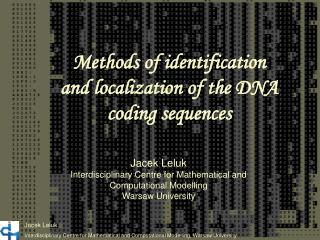Methods of identification and localization of the DNA coding sequences

# Methods of identification and localization of the DNA coding sequences - PowerPoint PPT Presentation

Methods of identification and localization of the DNA coding sequences. Jacek Leluk Interdisciplinary Centre for Mathematical and Computational Modelling Warsaw University. Jacek Leluk Interdisciplinary Centre for Mathematical and Computational Modelling, Warsaw University. Codon usage.I am the owner, or an agent authorized to act on behalf of the owner, of the copyrighted work described.
Download Presentation## Methods of identification and localization of the DNA coding sequences

An Image/Link below is provided (as is) to download presentation

Download Policy: Content on the Website is provided to you AS IS for your information and personal use and may not be sold / licensed / shared on other websites without getting consent from its author.While downloading, if for some reason you are not able to download a presentation, the publisher may have deleted the file from their server.

- - - - - - - - - - - - - - - - - - - - - - - - - - E N D - - - - - - - - - - - - - - - - - - - - - - - - - -
Presentation Transcript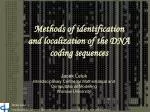Methods of identification

and localization of the DNA coding sequences

Jacek Leluk

Interdisciplinary Centre for Mathematical and Computational Modelling

Warsaw University

Jacek Leluk

Interdisciplinary Centre for Mathematical and Computational Modelling, Warsaw University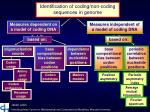Codon usage

Codon prototype

Markov models

Position asymmetry

Periodic asymmetry index

Identification of coding/non-coding sequences in genome

Measures dependent on a model of coding DNA

Measures independent of a model of coding DNA

based on:

based on:

oligonucleotide counts

base compositional bias between codon positions

dependence between nucleotide positions

base compositionalbias between codon positions

periodic correlation between nucleotide positions

Amino acid usage

Codon preference

Average mutual information

Hexamer usage

Fourier spectrum

Jacek Leluk

Interdisciplinary Centre for Mathematical and Computational Modelling, Warsaw University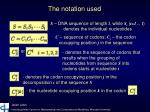or

The notation used

S – DNA sequence of length l, while Si(i=1 ... l) denotes the individual nucleotides

C – sequence of codons; Cj – the codon occupying position j in the sequence

- denotes the sequence of codons that results when the grouping of nucleotides from sequence Sinto codons starts at nucleotidei

,

- denotes the codon occupying position j in the decomposition i of the sequence S

[k]

- the nucleotide occupying position k in the codon

Jacek Leluk

Interdisciplinary Centre for Mathematical and Computational Modelling, Warsaw University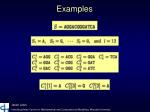Examples

Jacek Leluk

Interdisciplinary Centre for Mathematical and Computational Modelling, Warsaw University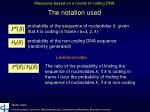Measures based on a model of coding DNA

The notation used

probability of the sequence of nucleotides S, given that S is coding in frame i (i=1, 2, 3)

probability of the non-coding DNA sequence (randomly generated)

Likelihood ratio

The ratio of the probability of finding the sequence of nucleotides S, if S is coding in frame i over the probability of finding the sequence of nucleotides S, if S is non-coding

Jacek Leluk

Interdisciplinary Centre for Mathematical and Computational Modelling, Warsaw University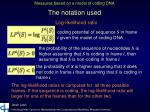Measures based on a model of coding DNA

The notation used

Log-likelihood ratio

coding potential of sequence S in frame i given the model of coding DNA

the probability of the sequence of nucleotides S is higher assuming that S is coding in frame i, than assuming that S is non-coding in frame i

the probability of S is higher assuming that S does not code in frame i than assuming that S is coding in frame i

The log-likelihood ratios is computed for all three possible frames. If the sequence is coding, the log-likelihood ratio will larger for one of the frames than for the other two.

Jacek Leluk

Interdisciplinary Centre for Mathematical and Computational Modelling, Warsaw University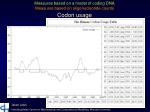Measures based on a model of coding DNA

Measures based on oligonucleotide counts

P0(C)=(1/64)m

Codon usage

frequency (probability) of codon C in the genes of the considered species (the codon usage table)

probability of finding the sequence of codons C knowing that C codes for a protein

probability of finding the non-coding sequence

Jacek Leluk

Interdisciplinary Centre for Mathematical and Computational Modelling, Warsaw University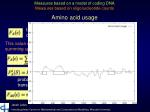Measures based on a model of coding DNA

Measures based on oligonucleotide counts

Amino acid usage

the observed probability of the amino acid encoded by codon C in the existing proteins

This value can be directly derived from a codon usage table by summing up the probabilities of synonymous codons

where

means c’ synonymous to c

probability of finding the amino acid sequence resulting of translating the sequence in coding open reading frame

frequency of the „non-coding amino acids”; nc – number of codons synonymous to C

Jacek Leluk

Interdisciplinary Centre for Mathematical and Computational Modelling, Warsaw University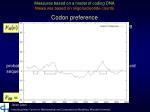Measures based on a model of coding DNA

Measures based on oligonucleotide counts

Codon preference

relative probability in coding regions of codon C among codons synonymous to C

probability of the sequence S encoding the particular amino acid sequence in frame i

In non-coding regions there is no preference between „synonymous codons”. Then:

probability of codon C in non-coding DNA

Jacek Leluk

Interdisciplinary Centre for Mathematical and Computational Modelling, Warsaw University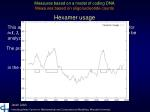Measures based on a model of coding DNA

Measures based on oligonucleotide counts

Hexamer usage

This approach is based on the hexamer usage table for i=1, 2, 3, ... , 4096. In this case there are six reading frames to be analyzed.

The probability of a sequence of hexanucleotides,

in the coding frame of a coding sequence is

Jacek Leluk

Interdisciplinary Centre for Mathematical and Computational Modelling, Warsaw UniversityMeasures based on a model of coding DNA

Measures based on base compositional bias between codon positions

Codon prototype

Let f(b,r) be the probability of nucleotide b at codon position r, as estimated from known coding regions. Then:

is the probability of codon c in coding regions, assuming independence between adjacent nucleotides

probability of for all triplets c in non-coding DNA

Example:

P2(S) and P3(S) are computed in similar way

Jacek Leluk

Interdisciplinary Centre for Mathematical and Computational Modelling, Warsaw UniversityMeasures based on a model of coding DNA

Measures based on dependence between nucleotide positions

Markov Models

In the Markov models the probability of a nucleotide at a particular codon position depends on the nucleotide(s) preceding it.

The Markov models of order 1 is the simplest of the Markov models.

The probability of a nucleotide depends only on the preceding nucleotide. In this case, the model of coding DNA is based on the probabilities of the four nucleotides at each codon position, depending on the nucleotide occurring at the preceding codon position (technically called the transition probabilities). Thus, instead of one single matrix, as in Codon Prototype, three 4x4 matrices (the transition matrices) are required, F1, F2, and F3, each one corresponding to a different codon position.

There are used Markov models of the order 1 to 5

Jacek Leluk

Interdisciplinary Centre for Mathematical and Computational Modelling, Warsaw UniversityMeasures independent of a model of coding DNA

Measures based on base compositional bias between codon positions

Position asymmetry

The goal is to measure how asymmetric is the distribution of nucleotides at the three triplet positions in the sequence.

the relative frequency of nucleotide b at codon r position in the sequence S, as calculated from one of the three decompositions of S in codons (any of them)

average frequency of nucleotide b at the three codon positions

asymmetry in the distribution of nucleotide b

Jacek Leluk

Interdisciplinary Centre for Mathematical and Computational Modelling, Warsaw UniversityMeasures independent of a model of coding DNA

Measures based on base compositional bias between codon positions

Position asymmetry (continued)

Position Asymmetry of the sequence

Jacek Leluk

Interdisciplinary Centre for Mathematical and Computational Modelling, Warsaw UniversityMeasures independent of a model of coding DNA

Measures based on periodic correlation between nucleotide positions

Periodic asymmetry index
• This approach considers three distinct probabilities:
• the probability Pin of finding pairs of the same nucleotide at distances k=2, 5, 8, ...
• the probability P1out of finding pairs of the same nucleotide at distances k=0, 3, 6, ...
• the probability P2out of finding pairs of the same nucleotide at distances k=1, 4, 7, ...

The tendency to cluster homogeneous di-nucleotides in a 3-base periodic pattern can be measured by the Periodic Asymmetry Index:

Jacek Leluk

Interdisciplinary Centre for Mathematical and Computational Modelling, Warsaw UniversityMeasures independent of a model of coding DNA

Measures based on periodic correlation between nucleotide positions

Average mutual information

absolute number of times when nucleotide i is followed by nucleotide j at a distance of k positions

probability that nucleotide i is followed by nucleotide j at a distance of k positions

Correlation between nucleotides i and j at a distance of k positions

where pi and pj are probabilities of nucleotide i and j occurrence in sequence S

Jacek Leluk

Interdisciplinary Centre for Mathematical and Computational Modelling, Warsaw UniversityMeasures independent of a model of coding DNA

Measures based on periodic correlation between nucleotide positions

Average mutual information (continued)

Mutual Information function

quantifies the amount of information that can be obtained from one nucleotide about another nucleotide at a distance k

Jacek Leluk

Interdisciplinary Centre for Mathematical and Computational Modelling, Warsaw UniversityMeasures independent of a model of coding DNA

Measures based on periodic correlation between nucleotide positions

Average mutual information (continued)

the in-frame mutual information at distances k=2, 5, 8, ...

the out-frame mutual information at distances k=0, 1, 3, 4, ...

Average Mutual Information

Jacek Leluk

Interdisciplinary Centre for Mathematical and Computational Modelling, Warsaw UniversityMeasures independent of a model of coding DNA

Measures based on periodic correlation between nucleotide positions

Fourier analysis

The partial spectrum of a DNA sequence S of length l corresponding to nucleotide b is defined as:

where Ub(Sj)=1 if Sj=b, and otherwise it is 0, and f is the discrete frequency, f =k/l, for k=1, 2, ... ,l/2

DNA coding regions reveal the characteristic periodicity of 3 as a distinct peak at frequency f =1/3

No such ``peak'' is apparent for non-coding sequences

Jacek Leluk

Interdisciplinary Centre for Mathematical and Computational Modelling, Warsaw UniversitySummary of results

Jacek Leluk

Interdisciplinary Centre for Mathematical and Computational Modelling, Warsaw UniversityJacek Leluk

Interdisciplinary Centre for Mathematical and Computational Modelling, Warsaw UniversityJacek Leluk

Interdisciplinary Centre for Mathematical and Computational Modelling, Warsaw UniversityThank you